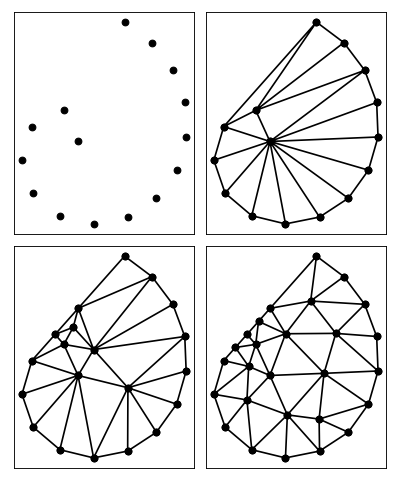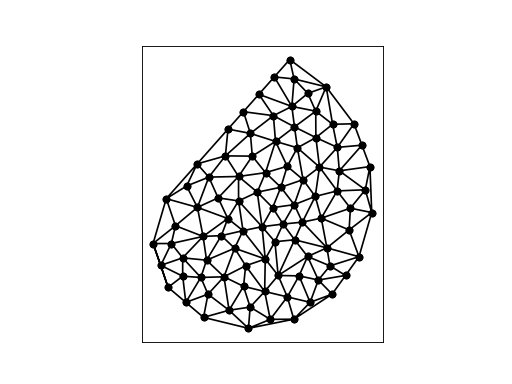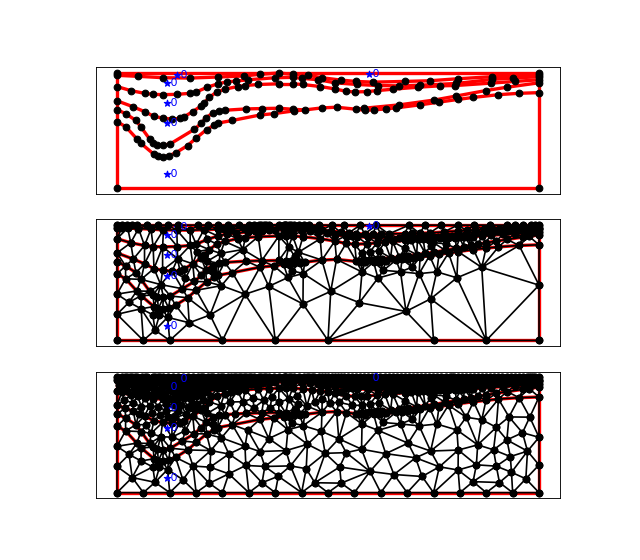# Quality Meshing: Angle and Size Constraints¶

Triangle generates a conforming constrained Delaunay triangulation whenever the -q, -a, or -u, switch is used. These switches set constraints on angles and triangle sizes in the mesh.

The -q switch sets a minimum angle constraint. A number may follow the q; otherwise, the default minimum angle is twenty degrees. For the vertex set spiral below, consider the differences among triangle spiral triangle -q spiral triangle -q32.5 spiralNote that the angle constraint does not apply to small angles between input segments; such angles cannot be removed.

The -a switch sets a maximum area constraint. There are three ways to use this switch. The first way is to specify a maximum area on the command line. The next example is a mesh in which no triangle has area greater than 0.2.

```triangulate(spiral,'a.2')
```The second manner of using the -a switch is applicable only when creating a new mesh from a PSLG. The file describing the PSLG itself contains area constraints, each of which is applied to a segment-bounded region; see the .poly file format for details. For an example, look at the last seven lines of la.poly, which describes a vertical cross section of soil in the Los Angeles Basin. Below is an illustration of the results when the -a switch is or is not invoked.

```triangulate(la,'pq')
triangulate(la,'pqa')
```